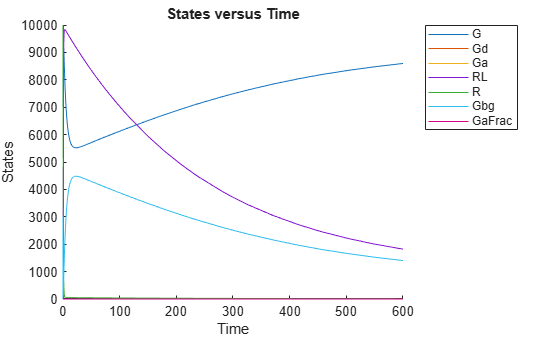# fitted

Return simulation results of SimBiology model fitted using least-squares regression

## Syntax

``````[yfit,parameterEstimates] = fitted(resultsObj)``````

## Description

example

``````[yfit,parameterEstimates] = fitted(resultsObj)``` returns simulation results `yfit` and parameter estimates `parameterEstimates` from a fitted SimBiology® model. TipUse this method to retrieve simulation results from the fitted model if you did not specify the second optional output argument that corresponds to simulation results when you first ran `sbiofit`. ```

## Examples

collapse all

This example uses the yeast heterotrimeric G protein model and experimental data reported by . For details about the model, see the Background section in Parameter Scanning, Parameter Estimation, and Sensitivity Analysis in the Yeast Heterotrimeric G Protein Cycle.

`sbioloadproject gprotein`

Store the experimental data containing the time course for the fraction of active G protein.

```time = [0 10 30 60 110 210 300 450 600]'; GaFracExpt = [0 0.35 0.4 0.36 0.39 0.33 0.24 0.17 0.2]';```

Create a `groupedData` object based on the experimental data.

```tbl = table(time,GaFracExpt); grpData = groupedData(tbl);```

Map the appropriate model component to the experimental data. In other words, indicate which species in the model corresponds to which response variable in the data. In this example, map the model parameter `GaFrac` to the experimental data variable `GaFracExpt` from `grpData`.

`responseMap = 'GaFrac = GaFracExpt';`

Use an `estimatedInfo` object to define the model parameter `kGd` as a parameter to be estimated.

`estimatedParam = estimatedInfo('kGd');`

Perform the parameter estimation.

`fitResult = sbiofit(m1,grpData,responseMap,estimatedParam);`

View the estimated parameter value of `kGd`.

`fitResult.ParameterEstimates`
```ans=1×3 table Name Estimate StandardError _______ ________ _____________ {'kGd'} 0.11307 3.4439e-05 ```

Suppose you want to plot the model simulation results using the estimated parameter value. You can either rerun the `sbiofit` function and specify to return the optional second output argument, which contains simulation results, or use the `fitted` method to retrieve the results without rerunning `sbiofit`.

`[yfit,paramEstim] = fitted(fitResult);`

Plot the simulation results.

`sbioplot(yfit);`## Input Arguments

collapse all

Estimation results, specified as an `OptimResults object`, `NLINResults object`, or vector of results objects which contains estimation results from running `sbiofit`.

## Output Arguments

collapse all

Simulation results, returned as a vector of `SimData` objects. The states reported in `yfit` are the states that were included in the `responseMap` input argument of `sbiofit` as well as any other states listed in the `StatesToLog` property of the runtime options (`RuntimeOptions`) of the SimBiology model.

Estimated parameter values, returned as a table. This argument is identical to the `resultsObj.ParameterEstimates` property.

 Yi, T-M., Kitano, H., and Simon, M. (2003). A quantitative characterization of the yeast heterotrimeric G protein cycle. PNAS. 100, 10764–10769.